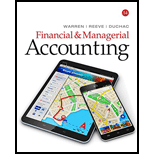# Break-even sales and cost-volume-profit chart Last year, I lever Inc. had sales of $500,000, based on a unit selling price of$250. The variable cost per unit was $175, and fixed costs were$75,000. The maximum sales within Hever Inc.’s relevant range are 2,500 units. Hever Inc. is considering a proposal to spend an additional $33,750 on billboard advertising during the current year in an attempt to increase sales and utilize unused capacity. Instructions 1. Construct a cost-volume-profit chart indicating the break-even sales for last year. Verify your answer, using the break-even equation. 2. Using the cost-volume-profit chart prepared in part (1), determine (A) the income from operations for last year and (B) the maximum income from operations that could have been realized during the year. Verify your answers using the mathematical approach to cost-volume-profit analysis. 3. Construct a cost-volume-profit chart indicating the break-even sales for the current year, assuming that a noncancellable contract is signed for the additional billboard advertising. No changes are expected in the unit selling price or other costs. Verify your answer, using the break-even equation. 4. Using the cost-volume-profit chart prepared in part (3), determine (A) the income from operations if sales total 2,000 units and (B) the maximum income from operations that could be realized during the year. Verify your answers using the mathematical approach to cost-volume-profit analysis.BuyFindarrow_forward ### Financial & Managerial Accounting 14th Edition Carl Warren + 2 others Publisher: Cengage Learning ISBN: 9781337119207 #### Solutions Chapter SectionBuyFindarrow_forward ### Financial & Managerial Accounting 14th Edition Carl Warren + 2 others Publisher: Cengage Learning ISBN: 9781337119207 Chapter 19, Problem 19.4APR Textbook Problem 40 views ## Break-even sales and cost-volume-profit chartLast year, I lever Inc. had sales of$500,000, based on a unit selling price of $250. The variable cost per unit was$175, and fixed costs were $75,000. The maximum sales within Hever Inc.’s relevant range are 2,500 units. Hever Inc. is considering a proposal to spend an additional$33,750 on billboard advertising during the current year in an attempt to increase sales and utilize unused capacity.Instructions1. Construct a cost-volume-profit chart indicating the break-even sales for last year. Verify your answer, using the break-even equation.2. Using the cost-volume-profit chart prepared in part (1), determine (A) the income from operations for last year and (B) the maximum income from operations that could have been realized during the year. Verify your answers using the mathematical approach to cost-volume-profit analysis.3. Construct a cost-volume-profit chart indicating the break-even sales for the current year, assuming that a noncancellable contract is signed for the additional billboard advertising. No changes are expected in the unit selling price or other costs. Verify your answer, using the break-even equation.4. Using the cost-volume-profit chart prepared in part (3), determine (A) the income from operations if sales total 2,000 units and (B) the maximum income from operations that could be realized during the year. Verify your answers using the mathematical approach to cost-volume-profit analysis.

1.

To determine

Cost-Volume-Profit Analysis: It is a method followed to analyze the relationship between the sales, costs, and the related profit or loss at various levels of units sold. In other words, it shows the effect of the changes in the cost and the sales volume on the operating income of the company.

To construct: a cost-volume-profit chart indicating the break-even sales for last year.

### Explanation of Solution

Construct a cost-volume-profit chart indicating the break-even sales for last year.

Figure (1)

The volume in units of sales is shown on the horizontal axis. The maximum relevant range is 2,500 units. The sales and the total costs (fixed cost and variable cost) in dollars is shown on the vertical axis. The maximum relevant range of sales and total costs is $700,000. The total sales line is drawn right upward by connecting the first point at$0 to the second point at $625,000 [2,500units×$250perunit] for 2,500 units sold (maximum relevant range on the horizontal axis)

2(A)

To determine
The income from operations for last year

2(B)

To determine
The maximum income from operations realized during the year.

2(A)

To determine

To verify: the answers using the mathematical approach to cost-volume-profit analysis.

2(B)

To determine
the maximum income from operations that could have been realized during the year.

3.

To determine

To construct: a cost-volume-profit chart indicating the break-even sales for the current year.

4(A)

To determine
the income from operations for sales 2,000 units

4(B)

To determine
The maximum income from operations that could have been realized during the year.

4(A)

To determine

To verify: the answers using the mathematical approach to cost-volume-profit analysis.

4(B)

To determine
the maximum income from operations that could have been realized during the year.

### Still sussing out bartleby?

Check out a sample textbook solution.

See a sample solution

#### The Solution to Your Study Problems

Bartleby provides explanations to thousands of textbook problems written by our experts, many with advanced degrees!

Get Started